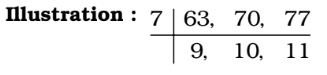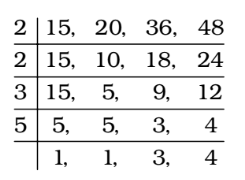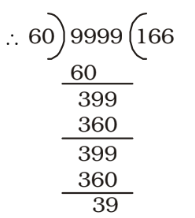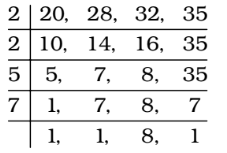## LCM and HCF

#### LCM and HCF

1. Three men step off together from the same spot. Their steps measure 63 cm, 70 cm and 77 cm respectively. The minimum distance each should cover so that all can cover the distance in complete steps is

1. We find LCM of 63, 70 and 77 cm.Required distance = LCM of 63, 70 and 77 cm.

##### Correct Option: C

We find LCM of 63, 70 and 77 cm.∴ LCM = 7 × 9 × 10 × 11 = 6930
Required distance = LCM of 63, 70 and 77 cm. = 6930

1. Find the least number which when divided separately by 15, 20, 36 and 48 leaves 3 as remainder in each case.

1. As we know that When a number is divided by a, b or c leaving same remainder ‘r’ in each case then that number must be k + r where k is LCM of a, b and c.
Required number = (LCM of 15, 20, 36 and 48) + 3∴ LCM ( k ) = 2 × 2 × 3 × 5 × 3 × 4 = 720

##### Correct Option: D

As we know that When a number is divided by a, b or c leaving same remainder ‘r’ in each case then that number must be k + r where k is LCM of a, b and c.
Required number = (LCM of 15, 20, 36 and 48) + 3∴ LCM ( k ) = 2 × 2 × 3 × 5 × 3 × 4 = 720
Here , r = 3
∴ Required number = k + r = 720 + 3 = 723

1. The greatest 4-digit number exactly divisible by 10, 15, 20 is

1. We know that Greatest n digit number which when divided by three numbers p,q,r leaves no remainder will be Required Number = (n – digit greatest number) – R , R is the remainder obtained on dividing greatest n digit number by L.C.M of p.q,r.
LCM of 10, 15 and 20 = 60
Largest 4-digit number = 9999##### Correct Option: B

We know that Greatest n digit number which when divided by three numbers p,q,r leaves no remainder will be Required Number = (n – digit greatest number) – R , R is the remainder obtained on dividing greatest n digit number by L.C.M of p.q,r.
LCM of 10, 15 and 20 = 60
Largest 4-digit number = 9999∴ Required number = 9999 – remainder = 9999 – 39 = 9960

1. The smallest perfect square divisible by each of 6, 12 and 18 is

1. The LCM of 6, 12 and 18 = 36 = 62

##### Correct Option: D

The LCM of 6, 12 and 18 = 36 = 62 = 36
Hence , required answer is 36.

1. The greatest number, which when subtracted from 5834, gives a number exactly divisible by each of 20, 28, 32 and 35, is

1. We find LCM of 20, 28, 32 and 35∴ LCM = 2 × 2 × 5 × 7 × 8 = 1120
∴ Required number = 5834 – LCM of 20, 28, 32 and 35

##### Correct Option: B

We find LCM of 20, 28, 32 and 35∴ LCM = 2 × 2 × 5 × 7 × 8 = 1120
∴ Required number = 5834 – LCM of 20, 28, 32 and 35
∴ Required number = 5834 – 1120 = 4714